Courses

# Basics Of Digital Electronics

## 10 Questions MCQ Test Topicwise Question Bank for GATE Electronics Engineering | Basics Of Digital Electronics

Description
This mock test of Basics Of Digital Electronics for Electronics and Communication Engineering (ECE) helps you for every Electronics and Communication Engineering (ECE) entrance exam. This contains 10 Multiple Choice Questions for Electronics and Communication Engineering (ECE) Basics Of Digital Electronics (mcq) to study with solutions a complete question bank. The solved questions answers in this Basics Of Digital Electronics quiz give you a good mix of easy questions and tough questions. Electronics and Communication Engineering (ECE) students definitely take this Basics Of Digital Electronics exercise for a better result in the exam. You can find other Basics Of Digital Electronics extra questions, long questions & short questions for Electronics and Communication Engineering (ECE) on EduRev as well by searching above.
QUESTION: 1

### The decimal equivalent of (101111.1101)2 is

Solution:

A binary number can be converted into decimal number by multiplying the binary numbers 1 or 0 by their weight and adding the product. Conversion of 101111 is done as follows: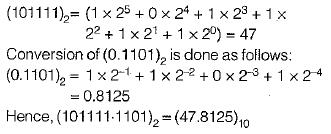QUESTION: 2

Solution:
QUESTION: 3

### Match List-l with List-ll and select the correct answer using the codes given below the lists: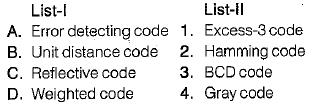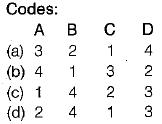Solution:
• “Hamming Code” provides a mathematical way to add one or more parity bits to a data character in order to detect and correct errors.
• “Gray code” is also called a “unit-distance code” because here two adjacent code numbers differ by only one bit.
• “Excess-3 code" is called a “reflective code” because here the code for 9 is the complement of the code for 0, 8 for 1,7 for 2, 6 for 3, and 5 for 4.
• “BCD code” is as “weighted code” or “8421 code” because it uses the binary number system to specify the decimal number 0 to 9.
QUESTION: 4

The following two numbers are converted into desired bases x and y respectively.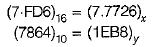The values of x and y are respectively

Solution: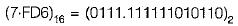The binary number is converted into octal number as follows: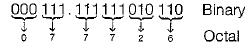Thus,

(7-FD6)16= (7.7726)8

On converting the decimal number (7864)10 into hexadecimal number we obtain the result (EB8)16 as follows: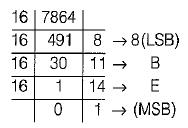Thus, (7864)10 = (1EB8)16

QUESTION: 5

If (100110) x (x)10 = (101010110)2, then the value of x will be equal to

Solution: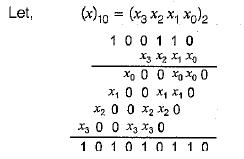From above multiplication, it is clear that,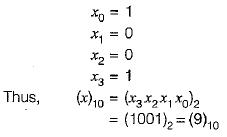QUESTION: 6

The range of numbers that can be represented using 6-bits in signed binary number representation is

Solution:

In signed binary number representation, the range of number that can be represented by an n -bit number is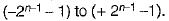For n = 6, the range is -31 to + 31.

QUESTION: 7

A decimal number (22)10 may be represented by the following ways:

Solution:

The binary equivalent of (22)10 = (10110)2 as follows: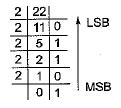∴  (22)10 = (10110)2

• The GRAY code of (10110)2 is obtained as follows: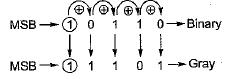Thus, (10110)2 = (11101)gray

• The octal equivalent of (22)10 = (26)8 obtained as follows: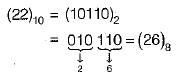• The hexadecimal equivalent of (22)10 obtained as follows: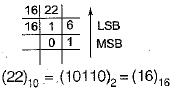QUESTION: 8

The 7-bit even parity Hamming code of the binary bits 0101 is

Solution:

Given,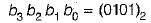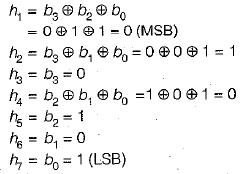∴ The required 7-bit even parity Hamming code is ( 0 1 0 0 1 0 1).

QUESTION: 9

If (2AC9)16 = (Z)7, then the value of Z is

Solution: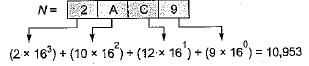Thus, (2AC9)16 - (10,953)10

Now, the decimal number is converted into the number of base 7 as follows: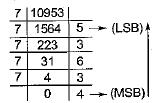Thus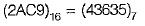QUESTION: 10

Assertion (A): Digital circuits are often called switching circuits.
Reason (R): Each type of digital circuit obeys a certain set of logic rules.

Solution:

Digital circuits are often called switching circuits, because the voltages in a digital circuit are assumed to be switching from one value to another instantaneously, that is, the transition time is assumed to be zero.
Reason is also a correct statement because digital circuit is a logic circuit, because each type of digital circuit obeys a certain set of logic rules. Hence, both assertion and reason are true but reason is not the correct explanation of assertion.